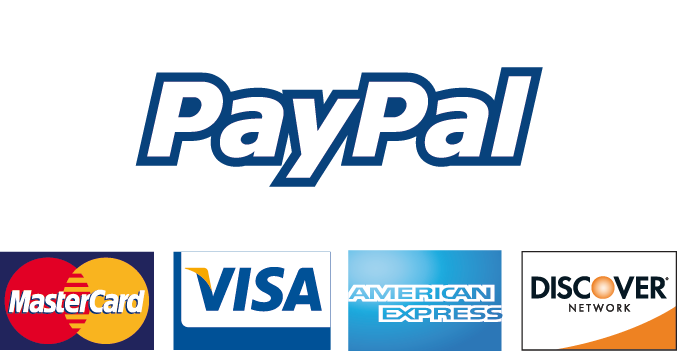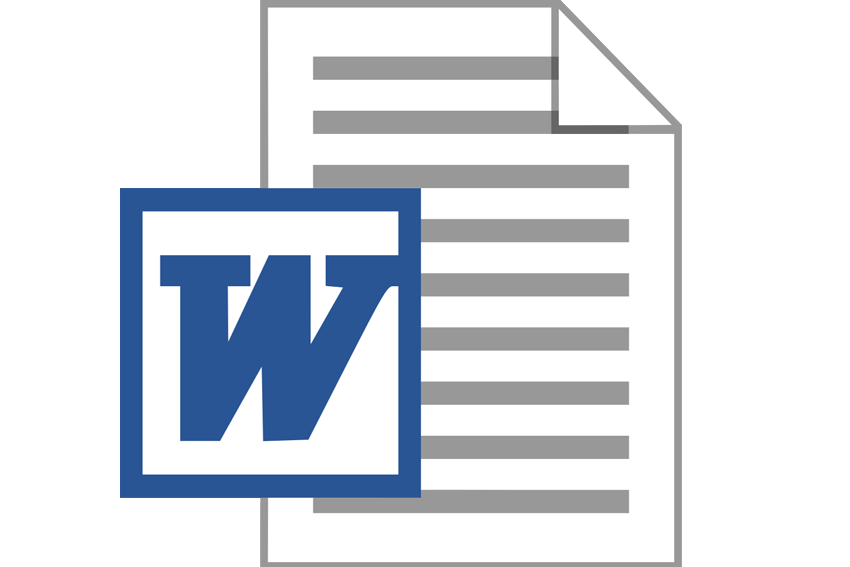We're Open
+44 7340 9595 39
+44 20 3239 6980

# 1) To calculate the dividend for the coming year, we need to multiply the last dividend by the expected dividend growth rate. And# Stocks and Bonds: Stock Valuation

INSTRUCTIONS:

The essay portion of the assignment should be not more than 2 pages long; no more.

There is also calculations in excel needed that is separate from the essay portion. Please placed the memo, essay and excel calculation is the order described in the instructions of the assignment. Please check the excel computations using the calculator located on the assignment instruction page. Use at least 2 references that are listed on the background page and the other two can be from another source. Please include the website URLs for all references and list the URLs on the reference page.

Module 2 - Case

Stocks and Bonds

Case Assignment

Stock Valuation

Mr. Hillbrandt is impressed with your ability to explain financial concepts so he asks for help with learning about stock valuation. Mr. Hillbrandt really liked the examples you provided last time (module 1), so it seems as if you need to sit down and create some relevant examples for this topic too. Below is some information that helps you brush up on the topic.

Read this article related to the intrinsic value of stock, paying special attention to the section entitled “Constant Growth Model”:

Alvarez, S. (2015). What is the intrinsic value of stock? Investopedia. Retrieved from http://www.investopedia.com/articles/basics/12/intrinsic-value.asp

Now, let’s work the following problem:

A company just paid an annual dividend of \$2.00 per share. Dividends are anticipated to grow at a rate of 8% per year forever. The stock’s beta is 1.5, the risk-free rate is 2.5%, and the expected return on the overall stock market is 7.5%. What’s the intrinsic value of the company’s common stock?

Using the formula:

Stock Price = D1 ÷ (k – g)

Where:

D1 = dividend for the coming year

k = required rate of return (NOTE: k must be greater than g)

g = growth rate of dividends

(Note: Decimals and not percentages must be used for the model to work)

1) To calculate the dividend for the coming year, we need to multiply the last dividend by the expected dividend growth rate. And so:

D1 = \$2.00 x 1.08 = \$2.16

2) Find the Market risk premium using the following formula:

Market risk premium = Expected return on stock - Risk free rate

= 7.5% - 2.5%

= 5%

3) Then, to find k, or the required rate of return, use the following formula:

k = risk free rate + [market risk premium x beta]

= 2.5% + (5% * 1.5)

= 10%

4) g = 8% (or 0.08) growth rate of dividends

5) Stock Price = D1 ÷ (k – g)

= \$2.16 ÷ (.10 - .08)

= \$2.16 ÷ .02

Now, work the following problems:

A company just paid an annual dividend of \$3.25 per share. Dividends are anticipated to grow at a rate of 5% per year forever. The stock’s beta is 1.2, the risk-free rate is 3.5%, and the expected return on the overall stock market is 5.5%. What’s the intrinsic value of the company’s common stock? ANSWER = \$379.17

A company just paid an annual dividend of \$2.35 per share. Dividends are anticipated to grow at a rate of 6.25% per year forever. The stock’s beta is 1.6, the risk-free rate is 4.25%, and the expected return on the overall stock market is 8.5%. What’s the intrinsic value of the company’s common stock? ANSWER = \$51.48

Required:

Part I consists of three questions.

Do the computations for the example below. Show the computations step by step, so Mr. Hillbrandt can easily follow your examples.

1. The company’s common stock dividends are anticipated to grow at a constant 5.5% growth rate per year going forward. The company just paid an annual dividend (that is, D-zero) of \$3 per share. What’s the intrinsic value of the stock based on the following required rates of return?

6%

8%

10%

12%

2. If the stock is currently selling for \$40 per share, is the stock a good buy? Interpret the results and justify your decision.

3. The company just paid an annual dividend of \$1.50 per share. Dividends are anticipated to grow at a stable rate of 10% per year forever. The stock’s beta is 1.2, the risk-free rate is 4%, and the expected return on the overall stock market is 11%. What is the intrinsic value of the company’s common stock?

Part II

Select one company that is a member of the Dow Jones Industrial Average. The listing is here:

http://finance.yahoo.com/q/cp?s=^DJI+Components

Apply the Dividend Discount Model and justify why you think that the stock is currently undervalued, overvalued, or fully valued. Please be sure to state your assumptions and justify your results. What is the relationship, if any, between stockholders’ wealth and financial decisions?

Computations (use Excel).

Use Excel to make the part I and II computations. Make sure you separate the two parts and organize the information, so Mr. Hillbrandt easily understands.

Memo (use Word).

Explain the concept and computations in part I to the CEO. Start with an introduction and end with a conclusion. Each of the four or five paragraphs should have a heading.

Short Essay - Part II (use Word).

Let’s look at this issue from an investor’s perspective and respond to the question above. Do research as needed and respond to the questions posed in part II.

Start with an introduction and end with a summary or conclusion. Use headings. Don’t forget to reference your sources. Maximum length of two pages.

Assignment Expectations

Each submission should include two files: (1) An Excel file; and (2) A Word document. The Word document shows the memo first and short essay last. Assume a knowledgeable business audience and use required format and length. Individuals in business are busy and want information presented in an organized and concise manner.

CONTENT:

Stocks and Bonds Name: Institution: Date: Part One Introduction The Dividend Discount Model is the most elegant and relatively simple way for individual investors to estimate the price they should be willing to pay for a stock, or determine whether a given stock is overvalued or undervalued. The Dividend discount model is based on the premise that the price of a stock should be equivalent to the sum of its current and future cash flow, after taking account of the time value of money. The Dividend Model formula is based on a basic valuation model that is a foundation for many other investing techniques. It combines the expected future cash flow and the time value of money. That is, Stock Price = the sum of the present value of all future dividends. Hypothetical Ex

...

## Price: £ 99

100% Plagiarism Free & Custom Written, Tailored to your instructions

### Details

• Title: 1) To calculate the dividend for the coming year, we need to multiply the last dividend by the expected dividend growth rate. And so:
• Price: £ 99
• Post Date: 2021-09-15T06:30:32+00:00
• Category: Essays
• No Plagiarism Guarantee
• 100% Custom Written

### Customer Reviews1) To calculate the dividend for the coming year, we need to multiply the last dividend by the expected dividend growth rate. And so:
Reviews: 5

### A masterpiece of assignment by Joshua , written on 2020-03-12

Writing is not my field. I take help from this website for my accounting assignment. The work is good and I scored good grades in it. Thank you from the bottom of my heart.
Reviews: 5

### A masterpiece of assignment by Mike , written on 2020-03-12

My order went 3 hours late and I also went mad. Then the customer support team calmed me down and offered me a flat discount of 50%. However, I did get my order and chilled out as the work was exactly what I requested. Next time I would place my order a bit sooner to save any trouble.